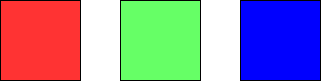# 用三种不同颜色为网格涂色

## 1931. 用三种不同颜色为网格涂色 (Hard)```输入：m = 1, n = 1

``````输入：m = 1, n = 2

```

```输入：m = 5, n = 5

```

• `1 <= m <= 5`
• `1 <= n <= 1000`

[动态规划]